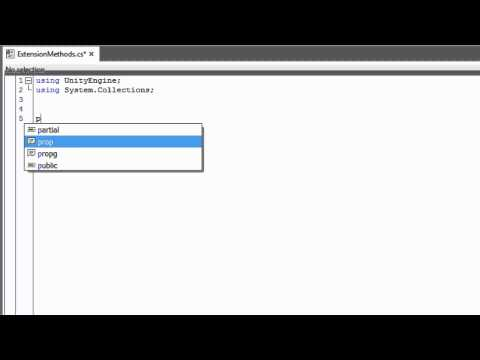# Extension Methods

#### 난이도: 중급

How to create, implement, and call an extension method.## Extension Methods

중급 Scripting

### Code snippet

``````using UnityEngine;
using System.Collections;

//It is common to create a class to contain all of your
//extension methods. This class must be static.
public static class ExtensionMethods
{
//Even though they are used like normal methods, extension
//methods must be declared static. Notice that the first
//parameter has the 'this' keyword followed by a Transform
//variable. This variable denotes which class the extension
//method becomes a part of.
public static void ResetTransformation(this Transform trans)
{
trans.position = Vector3.zero;
trans.localRotation = Quaternion.identity;
trans.localScale = new Vector3(1, 1, 1);
}
}``````
``````//Extension methods are not a feature of JavaScript. To gain this
//functionality you will need to use C#``````
``````import UnityEngine
import System.Collections

//It is common to create a class to contain all of your
//extension methods. This class must be static.
public static class ExtensionMethods:

//Even though they are used like normal methods, extension
//methods must be declared static. Notice that the first
//parameter has the 'this' keyword followed by a Transform
//variable. This variable denotes which class the extension
//method becomes a part of.
public static def ResetTransformation(trans as Transform):
trans.position = Vector3.zero
trans.localRotation = Quaternion.identity
trans.localScale = Vector3(1, 1, 1)``````

### Code snippet

``````using UnityEngine;
using System.Collections;

public class SomeClass : MonoBehaviour
{
void Start () {
//Notice how you pass no parameter into this
//extension method even though you had one in the
//method declaration. The transform object that
//this method is called from automatically gets
//passed in as the first parameter.
transform.ResetTransformation();
}
}``````
``````//Extension methods are not a feature of JavaScript. To gain this
//functionality you will need to use C#``````
``````import UnityEngine
import System.Collections

public class SomeClass(MonoBehaviour):

private def Start():
//Notice how you pass no parameter into this
//extension method even though you had one in the
//method declaration. The transform object that
//this method is called from automatically gets
//passed in as the first parameter.
transform.ResetTransformation()``````